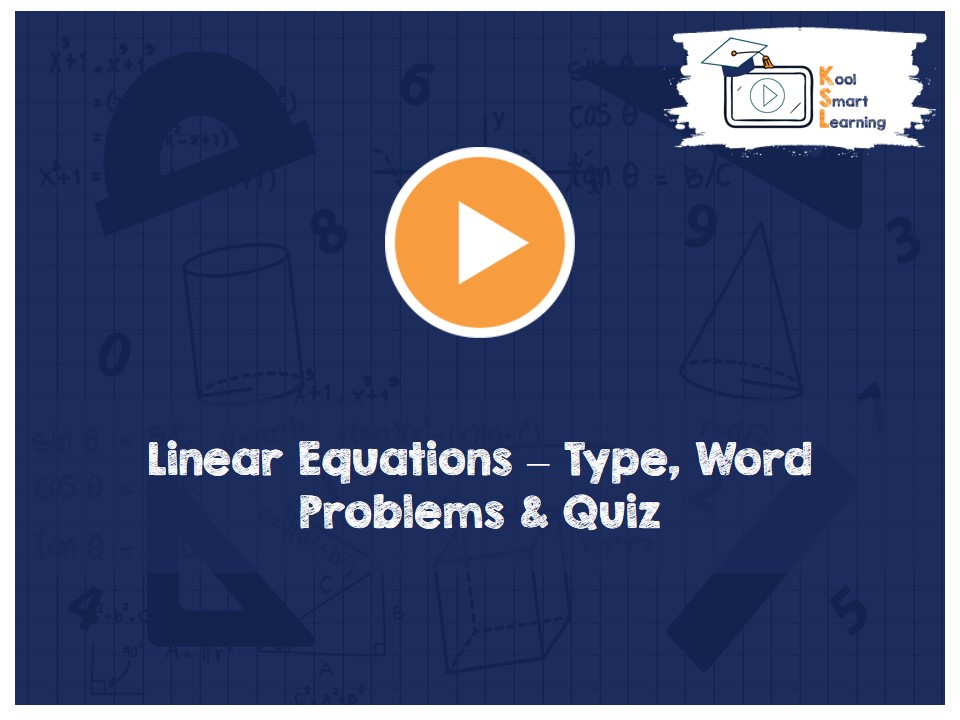At KoolSmartLearning, we intend to harness the power of online education to make learning easy.## Linear Equations – Type, Word Problems & Quiz

/  Linear Equations – Type, Word Problems & Quiz## Linear Equations – Type, Word Problems & Quiz

A video explaining linear equations in one variable. The video explains the equations reducible to the linear equations & solving them. It also covers how to convert the related word problems to linear equations in one variable & then solve it. The video also includes some questions & their solutions related to the linear equations in one variable.

More Related Videos